American Journal of Theoretical and Applied Statistics
Volume 4, Issue 5, September 2015, Pages: 414-419

Identifying the Limitation of Stepwise Selection for Variable Selection in Regression Analysis

Akinwande Michael Olusegun1, Hussaini Garba Dikko1, Shehu Usman Gulumbe2

1Department of Mathematics, Ahmadu Bello University, Zaria, Nigeria

2Department of Mathematics, Usman Danfodiyo University, Sokoto, Nigeria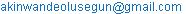(O. M. Akinwande)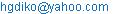(H. G. Dikko)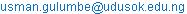(S. U. Gulumbe)

Akinwande Michael Olusegun, Hussaini Garba Dikko, Shehu Usman Gulumbe. Identifying the Limitation of Stepwise Selection for Variable Selection in Regression Analysis.American Journal of Theoretical and Applied Statistics.Vol.4, No. 5, 2015, pp. 414-419. doi: 10.11648/j.ajtas.20150405.22

Abstract: In application, one major difficulty a researcher may face in fitting a multiple regression is the problem of selecting significant relevant variables, especially when there are many independent variables to select from as well as having in mind the principle of parsimony; a comparative study of the limitation of stepwise selection for selecting variables in multiple regression analysis was carried out. Regression analysis in its bi-variate and multiple cases and stepwise selection (forward selection, backward elimination and stepwise selection) was employed for this study comparing the zero-order correlations and Beta () weights to give a clearer picture of the limitation of stepwise selection. Subsequently, from the comparisons, it was evident that including the suspected predictor (suppressor) variable that was not significant in the bi-variate case as suggested by the stepwise selection improved the beta weight of other predictors in the model and the overall predictability of the model as argued.

Keywords: Stepwise Selection, Suppression Effect, Regressor Weights, Correlation

Contents

1. Introduction

When selecting a set of study variables for regression analysis, researchers frequently test correlations between the outcome variables (i.e., dependent variables) and theoretically relevant predictor variables (i.e., independent variables) (Cohen, Cohen, West, & Aiken, 2013). In some instances, one or more of the predictor variables are uncorrelated with the outcome variable. This situation poses the question of whether researchers’ multiple regression analyses should exclude independent variables that are not significantly correlated with the dependent variable (Shanta & William, 2010). Questions such as this are routine, and our article provides a theoretical answer to these questions. In the multiple regression equations, suppressor variables increase the magnitude of regression coefficients associated with other independent variables or set of variables(Shanta & William, 2010). However, this situation leads us to the issue of variable selection procedures and methods.

Variable Selection

Often, theory gives only general direction as to which of a pool of explanatory variables (including transformed variables) should be included in the regression model. The actual set of predictor variables used in the final regression model must be determined by analysis of the data. Determining this subset is called the variable selection problem.(Conger, 1974)

Finding this subset of regressors (independent) variables involves two opposing objectives. First, the regression model should be as complete and realistic as possible (Darlington, 1968), every regressor that is even remotely related to the dependent variable to be included (a holistic view). Second, we want to include as few variables as possible (principle of parsimony) because each irrelevant regressor decreases the precision of the estimated coefficients and predicted values. Also, the presence of extra variables increases the complexity of data collection and model maintenance (Mendershausen, 1939). The goal of variable selection becomes one of parsimony: to achieve a balance between simplicity (as few regressors as possible) and fit (as many regressors as needed) (Lancaster, 1999). In ordinary least square regression analysis, many variable selection methods (processes) are available. Most of these selection rules depend mostly on the discretion of the researcher on which to apply (Loukas, 2005). However some of the variable selection methods are: forward selection, backward elimination and stepwise selection to mention but a few.

2. Methodology

A review of literatures related to the subject matter was undertaken to have a better understand the role and dynamic of suppressor variables. Also, a sample study was designed for the purpose of illustrating the possible disadvantages for not including such variables in a multiple regression analysis as well as the limitation of stepwise selection for variable selection.

Stepwise Selection

Stepwise selection is a combination of the forward and backward selection techniques (Yao, 2013). It was very popular at one time, stepwise regression is a modification of the forward selection so that after each step in which a variable was added, and all candidate regressor variables in the model are checked to see if their significance has been reduced below the specified tolerance level. If a non-significant variable is found, it is removed from the model.

Stepwise regression requires two significance levels: one for adding variables and one for removing variables. The cutoff probability for adding variables should be less than the cutoff probability for removing variables so that the procedure does not get into an infinite loop.

Theoretically, the stepwise process employs the F statistic in the partial F-test for its selection process. The test statistic for the stepwise process is denoted by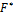and compares the Means Square of the Regressors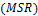and the Mean Square of the Error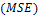for selecting relevant variables.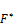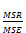(1)

The stepwise process begins by fitting a simple regression model for each of the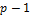potential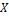variables: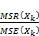(2)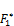=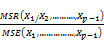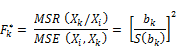Assuming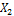is the variable entered in step 1, the stepwise process will fit all regression models with all variables whereis one of the pair. Therefore for such regression model, the partial F test statistic will be: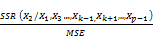(3)

If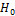holds, then~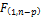. Large values ofleads to the conclusion of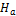. Recall that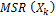= SSRmeasures the reduction in the total variation of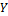associated with the use of variable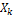. The variablewith the largestvalues is selected as the candidate variable for addition ifvalue exceeds a predetermined level. Thus, the variableis added otherwise the program terminates with novariable is considered sufficiently helpful to enter into the regression model (John, William, & Michael, 1983).

However, after careful considerations, the above mentioned procedures for variable selection has been found to mainly base its selection criterion on the correlation between the regressor(s) and the response variable. Which implies that the above mentioned variable selection process does not take into account the correlation within the regressors themselves that is (multicollinearity) which leads us to the idea that stepwise selection is limited in the sense that it is seemingly deficient in identifying predictor variable(s) that is significantly correlated with one or more predictor variables which is a severe draw back to the stepwise selection method.

Solely for the purpose of illustration, a simulated data was employed for this study. The data were generated using MINITAB statistical software. These data are 5 variables data, arbitrary names were also assigned to the variables which include: Grain Yield, Plant Heading, Plant Height, Tiller Count and Panicle Length respectively. A limitation of this study is that it is sometimes nearly impossible to have a set of data which has no correlation between them which informed our choice of a simulated data. However, having our objective in mind; that is, to show the limitation of stepwise selection in been able to select a variable with zero or near zero correlation with the response variable but significantly related to other predictors, we therefore require a set of predictor variables that exhibit the basic nature of the effect this work intends to show which is; the inability of stepwise selection to handle multicolinearity.

The statistical packages used for this study are MINITAB (version 14), and Microsoft Excel 2007. The choice of these packages is due to preference.

3. Analysis and Results

Quite a number of authors have proposed the understanding suppressor variables by evaluating regression weights (Conger, 1974) (Darlington, 1968). Instead of the regression weights, some researchers have preferred squared semipartial correlation of the suppressor variable in evaluating suppressor effect of a variable (Pedhazur, 1997). This current study intends to show the limitation of stepwise selection by evaluating the regressor weights and the general predictability of the regression model.

3.1. Hypothesis

We hypothesized that the Grain Yield of wheat if solely dependent on Plant Heading, Plant Height, Tiller Count and Panicle Length.

3.2. Measures

Five variables were picked from the wheat grain yield data: (a) Grain yield (b) Plant Heading (c) Plant Height (d) Tiller Count and (e) Panicle Length. Plant heading, Plant height, Tiller count and Panicle length were regarded as predictor (independent) variables while Grain yield was regarded as response (dependent) variable.

3.3. Results

The first step of analysis involves a Pearson zero order correlation of the five variables that is, Grain yield, plant heading, plant height, tiller count and panicle length. From table (1) below it can be clearly seen that Tiller count is not correlated with grain yield (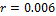) but is significantly related with plant heading (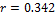), plant height (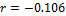) and panicle length (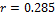) respectively. Also the correlation result shows that just two out of the four predictor variables are positively correlated with the outcome (response) variable (that is, plant heading and panicle length) therefore we might just conclude that the variables to be selected should be plant heading and panicle length leaving out plant height and tiller count.

4. Corellation

Table 1. Bi-variate Person zero order correlation.

 Grain Yield Plant Heading Plant Height Tiller Count Panicle Length Grain yield 1 Plant Heading 0.342344 1 P-Value 0.015 Plant Height -0.10686 0.124313 1 P-Value 0.460 0.390 Tiller Count 0.006782 0.176542 0.265493 1 P-Value 0.963 0.220 0.062 Panicle Length 0.285442 -0.05968 -0.07567 0.25715 1 P-Value 0.045 0.681 0.601 0.021

The second analytic step involved examining any potential adverse effect of correlated independent (predictor) variables. To this end, an investigation for the possibility of multi-collinearity among these four independent (predictor) variables was carried out. Also the correlation values between the four independent variables are:

Ÿ Plant heading and plant height, tiller count, panicle length (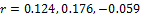)

Ÿ Plant height and tiller count and panicle length (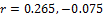)

Ÿ Tiller count and panicle length (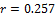)

More so, it can be clearly seen that indeed the Tiller Count variable is not significantly correlated with the Grain Yield (response) variable but it is correlated with the other predictor variables that is; Plant Heading, Plant Height and Panicle Length. This shows the presence of multicollinearity within the data.

The third analytic step is to employ the already existing methods of variable selection in regression analysis to get a clear picture of the potentially relevant variable(s) that will be suggested by the various methods of variable selection so as to further buttress our point.

4.1. Forward Selection

Stepwise Regression: Grain Yield versus Plant Heading, Plant Height, Tiller Count and Panicle Length.

Response is Grain Yield on 4 predictors, with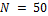Table 2. Forward Selection. Alpha-to-Enter: 0.5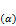.

 Step 1 2 Constant 255.4 140.5 Plant Heading 0.38 0.4 T-Value 2.52 2.78 P-Value 0.015 0.008 Panicle Length 0.34 T-value 2.37 P-Value 0.022 S 0.981 0.937 R-Sq 11.72 21.11 R-Sq (Adj) 9.88 17.75 Mallows C-P 5.7 2.2

From table 2 above, the forward selection process selected the plant heading and panicle length variable at 0.05 (α) as the significant variables to be included in the model as suggested by the correlation result in table 1 above with their corresponding p-values.

4.2. Backward Elimination

Stepwise Regression: Grain Yield versus Plant Heading, Plant Height, Tiller Count and Panicle Length.

Response is Grain Yield on 4 predictors, with.

Table 3. Backward Elimination. Alpha-to-Remove: 0.5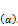Step 1 2 3 Constant 138.4 141.3 140.5 Plant Heading 0.41 0.42 0.40 T-value 2.76 2.88 2.78 P-value 0.008 0.006 0.008 Plant Height -0.14 -0.13 T-value -1.07 -1.00 P-value 0.291 0.321 Tiller Count 0.06 T-value 0.43 P-value 0.67 Panicle Length 0.34 0.33 0.34 T-value 2.31 2.29 2.37 P-value 0.026 0.027 0.022 S 0.946 0.937 0.937 R-Sq 23.11 22.80 21.11 R-Sq (Adj) 16.28 17.76 17.75 Mallows C-P 5 3.2 2.2

Table 4. Stepwise Selection Alpha to Enter: 0.05 and Remove: 0.05.

 Step 1 2 Constant 255.4 140.5 Plant Heading 0.38 0.4 T-value 2.52 2.78 P-value 0.015 0.008 Panicle Length 0.34 T-value 2.37 P-value 0.022 S 0.981 0.937 R-Sq 11.72 21.11 R-Sq (Adj) 9.88 17.75 Mallows C-P 5.7 2.2

Also, from table 3 above, the backward selection process selected the plant heading and panicle length variable at 0.05 (α) as the significant variables to be included in the model as suggested by the correlation result in table 1 above with their corresponding p-values.

4.3. Stepwise Selection

Stepwise Regression: Grain Yield versus Plant Heading, Plant Height, Tiller Count and Panicle Length.

Response is Grain Yield on 4 predictors, with.

Also, from table 4 above, the stepwise selection process selected the plant heading and panicle length variable at 0.05 (α) as the significant variables to be included in the model as suggested by the correlation result in table 1 above with their corresponding p-values.

From the three methods of variable selection (Tables 2, 3 and 4) (that is, forward selection, backward elimination and stepwise selection) above, it was deduce that plant heading and panicle length were the potentially relevant variables to be included in the model as suggested by the three variable selection methods. But it is against this backdrop that the limitation of stepwise selection is been argued considering the fact that the tiller count variable is positively correlated with the other predictors which is a case multicollinearity within the variables. To this end we are saying the Tiller Count variable should be included in the model.

The fourth analytic step is to run a regression of the variables both in the bi-variate and multiple variable cases to explicitly determine the significance of each variable in the bi-variate level.

5. Regression Analysis

5.1. The Bi-variate Case

5.1.1. Regression Analysis: Grain Yield Versus Plant Heading

The regression equation is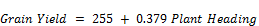(1.1)

Table 5. Summary of Regression Coefficients.

 Predictor Coef SE Coef T-value P-value Constant 255.44 37.55 6.80 0.000 Plant Heading 0.3790 0.1501 2.52 0.015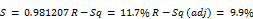Table 6. Analysis of Variance.

 Source Df Sum of Squares Mean Square F-ratio P-value Regression 1 6.1357 6.1357 6.37 0.015 Residual Error 48 46.2128 0.9628 Total 49 52.3485

Table 7. Summary of Regression Coefficients Table7.

 Predictor Coef SE Coef T-value P-value Constant 351.796 2.085 168.75 0.000 Plant Height -0.1044 0.1400 -0.75 0.460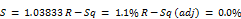5.1.2. Regression Analysis: Grain Yield Versus Plant Height

The regression equation is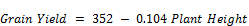(1.2)

Table 8. Analysis of Variance.

 Source Df Sum of Squares Mean Square F-ratio P-value Regression 1 0.599 0.599 0.56 0.460 Residual Error 48 51.750 1.078 Total 49 52.349

5.1.3. Regression Analysis: Grain Yield versus Tiller Count

The regression equation is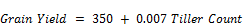(1.3)

Table 9. Summary of Regression Coefficients.

 Predictor Coef SE Coef T-value P-value Constant 350.213 0.735 476.55 0.000 Tiller Count 0.0065 0.1378 0.05 0.962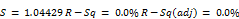Table 10. Analysis of Variance.

 Source Df Sum of Squares Mean Square F-ratio P-value Regression 1 0.002 0.002 0.00 0.962 Residual Error 48 52.346 1.091 Total 49 52.349

5.1.4. Regression Analysis: Grain Yield versus Panicle Length

The regression equation is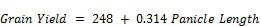(1.4)

Table 11. Summary of Regression Coefficients.

 Predictor Coef SE Coef T-value P-value Constant 248.14 49.49 5.01 0.000 Panicle Length 0.3141 0.1522 2.06 0.045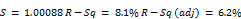Table 12. Analysis of Variance.

 Source Df Sum of Squares Mean Square F-ratio P-value Regression 1 4.264 4.264 4.26 0.045 Residual Error 48 48.085 1.002 Total 49 52.349

Result obtained from tables (5 to 12) the regression analysis in the bi-variate cases shows that the significant predictors among the four predictor variables are plant heading and panicle length. This implies that in the bi-variate level only plant heading and panicle length has significant relationship with the response (dependent) variable grain yield as suggested by the three variable selection methods above. The next step is to carry out the regression analysis in the multiple variable cases.

5.2. Multiple Variable Cases

5.2.1. Regression Analysis: Grain Yield Versus Plant Heading and Panicle Length

The regression equation is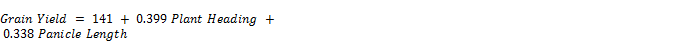(1.5)

Table 13. Summary of Regression Coefficients.

 Predictor Coef SE Coef T-value P-value Constant 140.55 60.39 2.33 0.024 Plant Heading 0.03993 0.1437 2.78 0.008 Panicle Length 0.3378 0.1428 2.37 0.022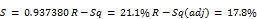Table 14. Analysis of Variance.

 Source Df Sum of Squares Mean Square F-ratio P-Value Regression 2 11.0505 5.5252 6.29 0.004 Residual Error 47 41.2981 0.8787 Total 49 52.3485

5.2.2. Regression Analysis: Grain Yield Versus Plant Heading, Tiller Count and Panicle Length

The regression equation is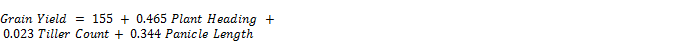(1.6)

Table 15. Summary of Regression Coefficients.

 Predictor Coef SE Coef T-value P-value Constant 154.66 61.39 2.27 0.028 Plant Heading 0.4648 0.1472 2.68 0.010 Tiller Count 0.0233 0.1312 0.18 0.860 Panicle Length 0.3444 0.1491 2.31 0.025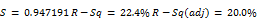Table 16. Analysis of Variance.

 Source Df Sum of Squares Mean Square F-ratio P-value Regression 3 11.0787 3.6929 4.12 0.011 Residual Error 46 41.2698 0.8972 Total 49 52.3485

6. Discussion

From the four regression analyses in the bi-variate case: Model 1.1, the outcome variable Grain Yield was regressed on the predictor variable Plant Heading, which was significant and accounted 11.7% of the variance in the outcome variable. Plant Heading was positive associated with grain yield (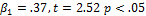). As Plant Heading increases by one unit Grain Yield increases by 37%.

In model 1.2, Grain yield versus Plant Height which was insignificant as expected. This account for only 1.1% of the variance in the outcome variable, Plant Height and Grain Yield were negatively associated (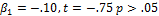), as Plant Height decreases by one unit Grain Yield Increases by - 11%.

In model 1.3, Grain Yield versus Tiller Count was insignificant as expected. Tiller Count and Grain Yield were not associated this does not account for any variability in the outcome variable (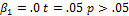).

In model 1.4, Grain Yield versus Panicle Length which was significant and accounted for 8.1% of the variance in the outcome variable. Panicle Length which was positively associated with Grain yield has (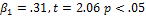). This implies as Panicle Length increases by one unit Grain Yield increases by 31%.

In model 1.5, Grain Yield versus Plant Heading and Panicle Length is significant as suggested by the stepwise variable selection method and it accounted for about 21.1% of the variance in the outcome variable. Plant Heading and Panicle Length were positively associated with the Grain Yield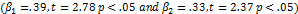More so, in model 1.6, Grain Yield versus Plant Heading, Tiller Count and Panicle Length was found also to be significant as against what the stepwise selection suggested. It accounted for about 22.4% of the variance in the outcome variable.

Furthermore, the inclusion of the Tiller Count variable in the model because of its correlation with the Plant Heading and Panicle Length variable improved the beta (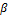) weight of Plant Heading from (0.399 to 0.465, p<.05) and that of Panicle Length from (0.338 to 0.344, p<.05). It also improved the overall predictability of the model as against the two predictor variable case.

7. Conclusion

Our ultimate objective in this paper was to call the attention of readers to the limitations of stepwise selection in for variable selection in regression analysis. The idea that a variable, which is unrelated to the dependent variable, should be retained not only for theoretical purposes but also to improve overall predictive power of the model is appealing. (Horst, The prediction of personal adjustment, 1941) recommended that researchers should retain a variable, even if it has near zero correlation with the response variable but have a significant correlation with other predictor variables. Further, other benefits accrue from including such a variable in multiple regression model(s).

Including such a variable will eliminate the danger of rejecting a true hypothesis as false (Shanta & Williams, 2010). As shown in this article, variables of this kind enrich the results of a multiple regression model, whereas premature elimination of such a variable reduces the predictive power of a model. Ideally, including this kind of variables in a model should be theory based and every regression model should include using a test for such an effects (Liebscher, 2012). This approach allows researchers to become aware of the limitations of stepwise selection in selection of potentially relevant variable to be included in a multiple regression model.

We have shown that it is possible to enhance the predictive power of a model by including a variable that was uncorrelated (or weakly correlated) with dependent variable, as long as the variable is correlated with other independent variable(s). Given this discussion of the limitations of stepwise selection, we suggest that researchers retain their list of independent variables, even if those variables are not significantly related with the dependent variable at the bivariate level, until they examine the variables for such an effect (suppression).

References

1. Cohen, J., Cohen, P., West, S. G., & Aiken, L. S. (2013). Applied multiple regression/correlation analysis for the behavioral sciences (Revised ed.). New York: Routledge.
2. Conger, A. J. (1974). A revised definition for suppressor variables: A guide to their identification and interpretation. Educational and Psychological Measurement , 35-46.
3. Darlington, R. B. (1968). Multiple regression in psychological research and practice. Psychological Bulletin , 161-182.
4. Horst, P. (1941). The prediction of personal adjustment. Social Science Research Council Bulletin , 431-436.
5. John, N., William, W., & Michael, H. K. (1983). Stepwise Selection. In N. John, W. William, & H. K. Michael, Applied Linear regression Models (pp. 430-434). Illinois: Richard D Irwin Inc.
6. Lancaster, B. P. (1999). Defining and interpreting suppressor effects: Advantages and limitations. Southwest Educational Research Association, San Antonio , 1-21.
7. Liebscher, G. (2012). A Universal Selection Method in Linear Regression Models. Open Journal of Statistics , 153-162.
8. Loukas, A. P. (2005). Early adolescent social and overt aggression: Examining the roles of social anxiety and maternal psychological control. Journal of Youth and Adolescence , 335-345.
9. Mendershausen, H. (1939). Clearing variates in confluence analysis. Journal of the American Statistical Association , 93-105.
10. Nathans, L. L. (2012). Interpreting Multiple Linear Regression: A Guidebook of Variable Importance. Practical Assessment, Research & Evaluation , 17, 123-136.
11. Pedhazur, E. J. (1997). Multiple regression in behavioral research. New York: Holt, Rinehart & Winston.
12. Shanta, P., & Williams, E. (2010). Suppressor Variables in Social Work Research: Ways to Identify in Multiple Regression Models. Journal of the Society for Social Work and Research , 28-40.
13. Shieh, G. (2006). Suppression situations in multiple linear regression. Educational and Psychological Measurement , 435-447.
14. Yao, J. (2013). Precision Analysis and Parameter Inversion in the Stepwise Deployment of a Mixed Constellation. Open Journal of Statistics , 390-397.

 Contents 1. 2. 3. 3.1. 3.2. 3.3. 4. 4.1. 4.2. 4.3. 5. 5.1. 5.2. 6. 7.
Article ToolsAbstractPDF(249K)